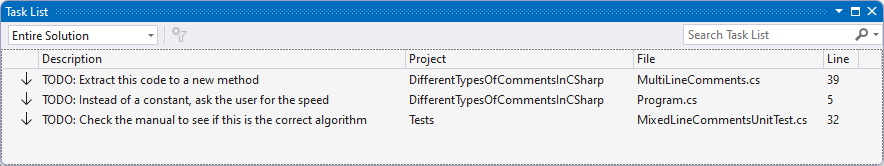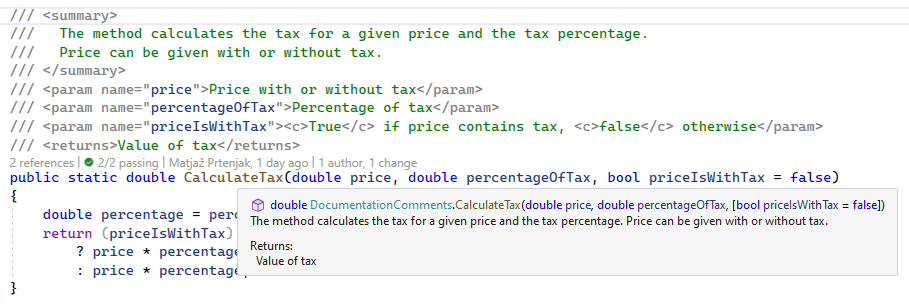The compiler will compile any syntactically correct code into an intermediate language, and after that, the .NET runtime will execute this IL code on the client computer. The computer will run any algorithm we write, no matter how wrong it is. To a computer, it is all the same; it does what we tell it to do.

We, as programmers, need to understand the code, and we should write code so that humans can read it. An essential part of writing the code is also commenting on it. We comment on the code for us, for humans/programmers, not for computers. And there is a fine line between code without comments and code with too many comments.

But to start, let us see how exactly we can comment C# code.

Single-line comments are the easiest, and every computer language knows them. With two consecutive slashes `//`, we tell the C# compiler that from this point on, the text is not intended for compiling and can be ignored:

```// Gravity 9.81 meters per second squared
const double GRAVITY = 9.81;

var time = 5; // time of falling is 5 seconds

// To calculate the speed at which the object will hit the floor,
// we have to use the simple formula:
// speed = gravity * time of falling
var speed = GRAVITY * time;```

We use single-line comments in three different places.

The first example represents the comment that takes the whole line, so there is only the comment in that entire line.

Next, commenting on just part of the line is possible. First, there is the C# code, and then there is a comment on what 5 means.

The last example shows that it is also possible to have comment after comment in every line. But for these particular cases, we also have so-called multi-line comments that we will see in the next paragraph.

After `//` everything is a comment until the end of the line, and any C# code in the comment will be ignored:

`var time = 5; // var speed = 10;`

Here the variable speed will not exist because it is in the comment, and the compiler will ignore everything in the comments.

When we are developing an app, we can spot a bug or get an idea or something, but we don’t want to break out of “the zone”. Or we are looking at the program and getting an idea of what else we could/should do.

In this situation, we can use a special type of TODO comment:

```// Gravity 9.81 meters per second squared
const double GRAVITY = 9.81;

// TODO: Instead of a constant, ask the user for the speed
var time = 5; // time of falling is 5 seconds```

We have used the TODO comment to remind ourselves that in the future we should repair our program so it will ask the user and not use a constant value for the speed.

Then we could search all our code base for `"// TODO"` strings, which would work. We would find all the comments on our to-do list. But Visual Studio has an even better solution which we can see by using the menu option `View / Task List` which will open a new Task List window:Visual Studio has a special window to see all about our to-do items. We can see the text, location, project, and source file. If we double-click an element, Visual Studio will position us on the exact spot in the code.

In the previous section, we saw three consecutive single-line comments, which are syntactically correct, but we have to type `//` at the beginning of every line.

So if we need more than one line of comments, we can use particular comment types `/*` and `*/`. Everything between these special characters is a comment, and the compiler will ignore it. So instead of single-line comments (`//`), we can use multi-line (`/*` `*/`) comments:

```// Gravity 9.81 meters per second squared
const double GRAVITY = 9.81;

var time = 5; // time of falling is 5 seconds

/*
To calculate the speed at which the object will hit the floor,
we have to use the simple formula:
speed = gravity * time of falling
*/
var speed = GRAVITY * time;```

This is the same code, but we use multi-line comments instead of single-line ones.

If we use multi-line comments, we need to close them with `*/`. The compiler will ignore everything until it sees `*/`.

This example also shows why we have two types of comments. This is more convenient for us.

We will use single-line comments when we want to say everything from that point (`//`) up until the end of the line is a comment. But if we want to extend the comment(s) beyond the end of the line, we will use the combination of (`/*`) and (`*/`) to mark the beginning and the end of the comments.

Modern computer languages also have special structured comments, and documentation programs can use that structure to create code documentation automatically.

Now that we know everything about single-line and multi-line comments, documentation comments are easy to understand. We can use triple slashes (`///`) or the combination (`/**`) to start and (`*/`) to end the documentation comment:

```/// <summary>
///   This class represents a Car.
/// </summary>
public class Car {}

/**
<summary>
This class represents a Giraffe.
</summary>
*/
public class Giraffe {}```

The second comment type `/**` and `*/` is rarely used. In the C# code, we will likely find these documentation comments (`///`) because Visual Studio greatly supports them.

If we have a `CalculateTax` method:

```public static double CalculateTax(double price, double percentageOfTax, bool priceIsWithTax = false)
{
double percentage = percentageOfTax / 100;
return (priceIsWithTax)
? price * percentage / (1 + percentage)
: price * percentage;
}```

And then, we type `///` right above that method:

```///
public static double CalculateTax(double price, double percentageOfTax, bool priceIsWithTax = false)
{
...
}```

Visual Studio will automatically check the method signature and prepare documentation comments:

```/// <summary>
///
/// </summary>
/// <param name="price"></param>
/// <param name="percentageOfTax"></param>
/// <param name="priceIsWithTax"></param>
/// <returns></returns>
public static double CalculateTax(double price, double percentageOfTax, bool priceIsWithTax = false)
{
double percentage = percentageOfTax / 100;
return (priceIsWithTax)
? price * percentage / (1 + percentage)
: price * percentage;
}```

```/// <summary>
///   The method calculates the tax for a given price and the tax percentage.
///   Price can be given with or without tax.
/// </summary>
/// <param name="price">Price with or without tax</param>
/// <param name="percentageOfTax">Percentage of tax</param>
/// <param name="priceIsWithTax"><c>True</c> if price contains tax, <c>false</c> otherwise</param>
/// <returns>Value of tax</returns>```

We have successfully documented our method. We can now generate HTML documentation, but not only that. If we position our mouse on the method’s name, Visual Studio will immediately display the help just as for any other C# method, which is pretty cool:Visual Studio is displaying our documentation comments as help for using our new method.

### Different Tags in Documentation Comments

In documentation comments, we can use different tags for different purposes. Up until now, we used a few tags `<summary>`, `<param>`, and `<returns>`. But of course, there are others as well:

TagDescription
cSet text in a code-like font
codeSet one or more lines of source code or program output
exampleIndicate an example
exceptionIdentifies the exceptions a method can throw
paramDescribe a parameter for a method or constructor
paramrefIdentify that a word is a parameter name
returnsDescribe the return value of a method
summaryDescribe a type or a member of a type
valueDescribe a property

We saw that Visual Studio could immediately use the documentation comments. Still, they are even more helpful if we use one of the external tools, like Doxygen, and generate documentation in HTML, PDF, or any other format that the concrete program supports.

## Should We Write Comments in Our Code?

Yes! When we write code, we know what we are doing. We know what each variable represents and what each method does. But after a while, we will forget and need something to remind us of what the code does. But not only us. What if some other developer works on our code? They will also need some guidance that comments can provide.

Nevertheless, we should always write self-explanatory code.

```// add ten
value = value + 10

int i; // i is a counter

// looping through messages
for(i = 0; i < 10; ++i) { ... }```

None of that code needs comments. The first comment is useless; any developer can see that we are adding 10 to some variable.

The second and third comments represent bad coding or the so-called “code smell”. These comments are here because the intent of the code is not visible.

So, we can make this code better without the comments:

```int counter;

foreach(var message in messages) { ... }```

This code does not need comments, as the intent is obvious. If we follow some simple rules, we only need a few comments.

### Good Commenting Practices

The first and most difficult rule is to use appropriate names for variables, methods, classes, and namespaces. This sounds easy but is quite complex in reality.

The second rule is to make methods small, not more than a few lines. If the method becomes too big, split it into two or more methods. The code can again become self-explanatory by naming this new method right (first rule).

So by following these two rules, there is no need to comment on every single line of code, but we should write comments for methods and classes. Even there, we should be conservative and write only comments introducing new information not seen in the code. We do not need to comment on a method named `FindProductById`, as each developer should know what and how this method works.

## Conclusion

In this article, we learned about different types of comments in C#. We saw that we can use three different kinds of comments. For small comments that fit into one line, we should use single-line comments `//`. If comments are longer than one line, we should use multi-line comments using `/*` and `*/`.

Then there is the third type of comments, documentation comments that fulfill two roles. They are regular comments in the code, but we can also automatically generate code documentation.

And at the end, we discussed a few things about when to write comments and what to comment on.